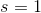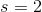# ISEE Upper Level Quantitative : How to find the area of a hexagon

## Example Questions

### Example Question #1 : Hexagons

Which is the greater quantity?

(a) The area of a regular hexagon with sidelength 1

(b) The area of an equilateral triangle with sidelength 2

It is impossible to tell from the information given

(b) is greater

(a) and (b) are equal

(a) is greater

A regular hexagon with sidelengthcan be seen as a composite of six equilateral triangles, each with sidelength. Since area is in direct proportion to the square of the sidelength, the area of the equilateral triangle with sidelengthis equal to that of four of those triangles. This makes the hexagon greater in area, and it makes (a) the greater quantity.